Balanced vs. Unbalanced Forces - Directions

The Balanced vs. Unbalanced Force Concept Builder is comprised of 44 questions. The questions are divided into 12 different question groups. Questions in the same group are rather similar to one another. The Concept Builder is coded to select at random a question from each group until a student is successful with that group of questions.

There are three levels of difficulty by which the student can progress through the Concept Builder. Those three difficulty levels are differentiated as follows:

• Apprentice Level: Groups 1-4
• Masters Level: Groups 1-8
• Wizard Level: Groups 1-12

The questions from each group are shown below. Teachers are encouraged to view the questions in order to judge which level of difficulty is most appropriate for their classes. We recommend providing students two or more options. For instance, it would be profitable for a student to complete the Concept Builder at the Apprentice Level in order to gain some confidence and then to be challenged to complete the activity at the Masters Level.

The Physics Classroom grants teachers and other users the right to print these questions for private use. Users are also granted the right to copy the text and modify it for their own use. However, this document should not be uploaded to other servers for distribution to and/or display by others. The Physics Classroom website should remain the only website or server from which the document is distributed or displayed. We also provide a PDF that teachers can use under the same conditions. We have included a link to the PDF near the bottom of this page.

Group #1
1. The diagram below represents the forces acting upon a rightward-moving object. Each arrow represents a force; the length of the arrow represents the strength of the force.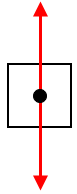A. Is this object speeding up, slowing down, or moving at a constant speed?
B. Is this object experiencing balanced forces or unbalanced forces?

2. The diagram below represents the forces acting upon a leftward-moving object. Each arrow represents a force; the length of the arrow represents the strength of the force.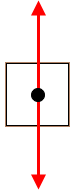A. Is this object speeding up, slowing down, or moving at a constant speed?
B. Is this object experiencing balanced forces or unbalanced forces?

3.    The diagram below represents the forces acting upon a rightward-moving object. Each arrow represents a force; the length of the arrow represents the strength of the force.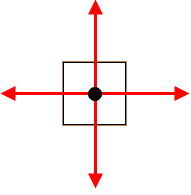A. Is this object speeding up, slowing down, or moving at a constant speed?
B. Is this object experiencing balanced forces or unbalanced forces?

4.    The diagram below represents the forces acting upon a leftward-moving object. Each arrow represents a force; the length of the arrow represents the strength of the force.A. Is this object speeding up, slowing down, or moving at a constant speed?
B. Is this object experiencing balanced forces or unbalanced forces?

Group #2
5.    The diagram below represents the forces acting upon a rightward-moving object. Each arrow represents a force; the length of the arrow represents the strength of the force.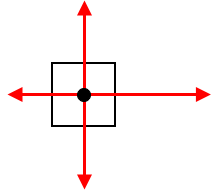A. Is this object speeding up, slowing down, or moving at a constant speed?
B. Is this object experiencing balanced forces or unbalanced forces?

6.    The diagram below represents the forces acting upon a leftward-moving object. Each arrow represents a force; the length of the arrow represents the strength of the force.A. Is this object speeding up, slowing down, or moving at a constant speed?
B. Is this object experiencing balanced forces or unbalanced forces?

7.    The diagram below represents the forces acting upon a rightward-moving object. Each arrow represents a force; the length of the arrow represents the strength of the force.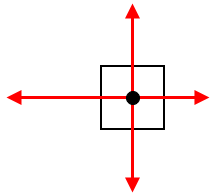A. Is this object speeding up, slowing down, or moving at a constant speed?
B. Is this object experiencing balanced forces or unbalanced forces?

8.    The diagram below represents the forces acting upon a leftward-moving object. Each arrow represents a force; the length of the arrow represents the strength of the force.A. Is this object speeding up, slowing down, or moving at a constant speed?
B. Is this object experiencing balanced forces or unbalanced forces?

Group #3
9.    The diagram below represents the forces acting upon a rightward-moving object. Each arrow represents a force; the length of the arrow represents the strength of the force.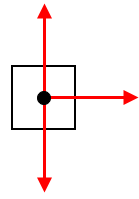A. Is this object speeding up, slowing down, or moving at a constant speed?
B. Is this object experiencing balanced forces or unbalanced forces?

10.  The diagram below represents the forces acting upon a leftward-moving object. Each arrow represents a force; the length of the arrow represents the strength of the force.A. Is this object speeding up, slowing down, or moving at a constant speed?
B. Is this object experiencing balanced forces or unbalanced forces?

11.  The diagram below represents the forces acting upon a rightward-moving object. Each arrow represents a force; the length of the arrow represents the strength of the force.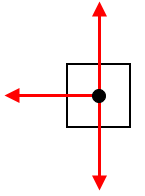A. Is this object speeding up, slowing down, or moving at a constant speed?
B. Is this object experiencing balanced forces or unbalanced forces?

12.  The diagram below represents the forces acting upon a leftward-moving object. Each arrow represents a force; the length of the arrow represents the strength of the force.A. Is this object speeding up, slowing down, or moving at a constant speed?
B. Is this object experiencing balanced forces or unbalanced forces?

Group #4
13.  The diagram below represents the forces acting upon an upward-moving object. Each arrow represents a force; the length of the arrow represents the strength of the force.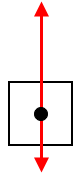A. Is this object speeding up, slowing down, or moving at a constant speed?
B. Is this object experiencing balanced forces or unbalanced forces?

14.  The diagram below represents the forces acting upon a downward-moving object. Each arrow represents a force; the length of the arrow represents the strength of the force.A. Is this object speeding up, slowing down, or moving at a constant speed?
B. Is this object experiencing balanced forces or unbalanced forces?

15.  The diagram below represents the forces acting upon an upward-moving object. Each arrow represents a force; the length of the arrow represents the strength of the force.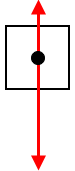A. Is this object speeding up, slowing down, or moving at a constant speed?
B. Is this object experiencing balanced forces or unbalanced forces?

16.  The diagram below represents the forces acting upon a downward-moving object. Each arrow represents a force; the length of the arrow represents the strength of the force.A. Is this object speeding up, slowing down, or moving at a constant speed?
B. Is this object experiencing balanced forces or unbalanced forces?

Group #5
17.  The motion of a rightward-moving object is represented by a position-time graph. (Consider the positive direction to be to the right.)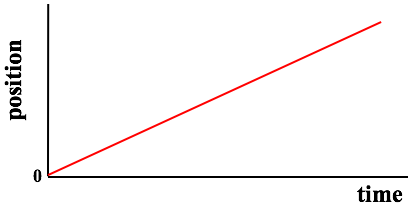A. Is this object speeding up, slowing down, or moving at a constant speed?
B. Is this object experiencing balanced forces or unbalanced forces?

18.  The motion of a leftward-moving object is represented by a position-time graph. (Consider the positive direction to be to the right.)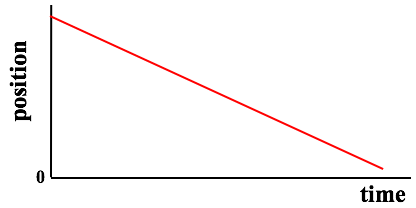A. Is this object speeding up, slowing down, or moving at a constant speed?
B. Is this object experiencing balanced forces or unbalanced forces?

Group #6
19.  The motion of a rightward-moving object is represented by a position-time graph. (Consider the positive direction to be to the right.)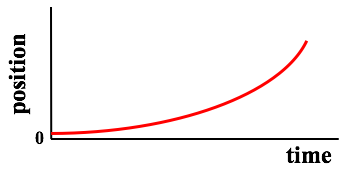A. Is this object speeding up, slowing down, or moving at a constant speed?
B. Is this object experiencing balanced forces or unbalanced forces?

20.  The motion of a rightward-moving object is represented by a position-time graph. (Consider the positive direction to be to the right.)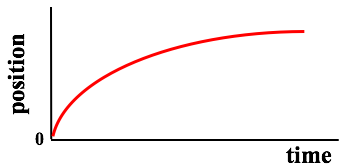A. Is this object speeding up, slowing down, or moving at a constant speed?
B. Is this object experiencing balanced forces or unbalanced forces?

21   The motion of a leftward-moving object is represented by a position-time graph. (Consider the positive direction to be to the right.)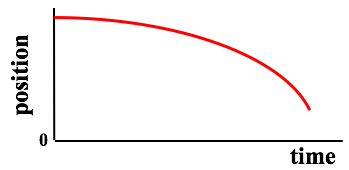A. Is this object speeding up, slowing down, or moving at a constant speed?
B. Is this object experiencing balanced forces or unbalanced forces?

22.  The motion of a leftward-moving object is represented by a position-time graph. (Consider the positive direction to be to the right.)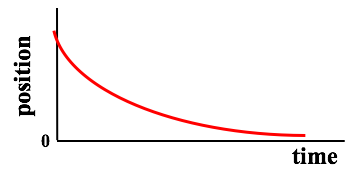A. Is this object speeding up, slowing down, or moving at a constant speed?
B. Is this object experiencing balanced forces or unbalanced forces?

Group #7
23.  The motion of a rightward-moving object is represented by the dot diagram below.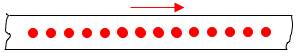A. Is this object speeding up, slowing down, or moving at a constant speed?
B. Is this object experiencing balanced forces or unbalanced forces?

24.  The motion of a leftward-moving object is represented by the dot diagram below.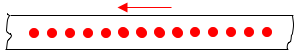A. Is this object speeding up, slowing down, or moving at a constant speed?
B. Is this object experiencing balanced forces or unbalanced forces?

Group #8
25.  The motion of a rightward-moving object is represented by the dot diagram below.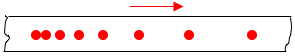A. Is this object speeding up, slowing down, or moving at a constant speed?
B. Is this object experiencing balanced forces or unbalanced forces?

26.  The motion of a rightward-moving object is represented by the dot diagram below.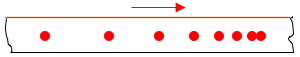A. Is this object speeding up, slowing down, or moving at a constant speed?
B. Is this object experiencing balanced forces or unbalanced forces?

27.  The motion of a leftward-moving object is represented by the dot diagram below.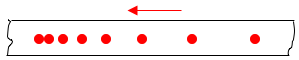A. Is this object speeding up, slowing down, or moving at a constant speed?
B. Is this object experiencing balanced forces or unbalanced forces?

28.  The motion of a leftward-moving object is represented by the dot diagram below.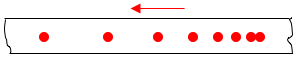A. Is this object speeding up, slowing down, or moving at a constant speed?
B. Is this object experiencing balanced forces or unbalanced forces?

Group #9
29.  The motion of an object is represented by a velocity-time graph.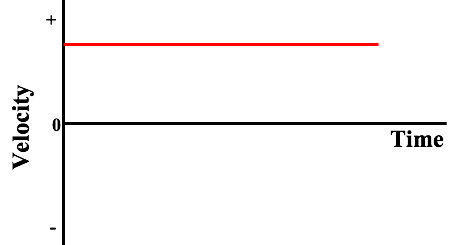A. Is this object speeding up, slowing down, or moving at a constant speed?
B. Is this object experiencing balanced forces or unbalanced forces?

30.  The motion of an object is represented by a velocity-time graph.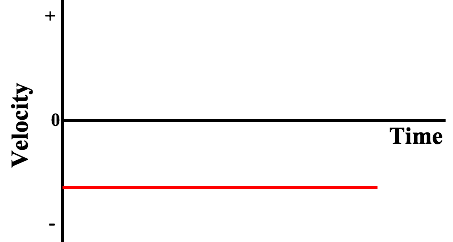A. Is this object speeding up, slowing down, or moving at a constant speed?
B. Is this object experiencing balanced forces or unbalanced forces?

Group #10
31.  The motion of an object is represented by a velocity-time graph.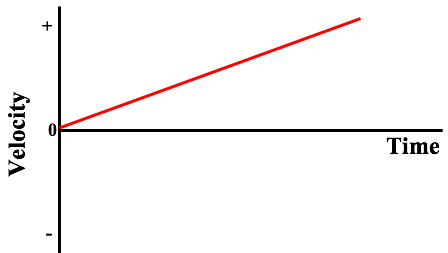A. Is this object speeding up, slowing down, or moving at a constant speed?
B. Is this object experiencing balanced forces or unbalanced forces?

32.  The motion of an object is represented by a velocity-time graph.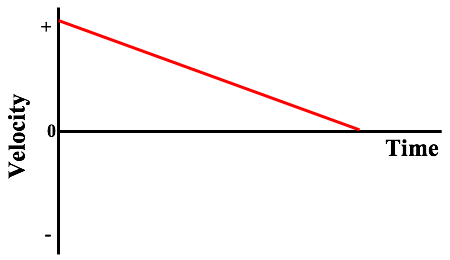A. Is this object speeding up, slowing down, or moving at a constant speed?
B. Is this object experiencing balanced forces or unbalanced forces?

33.  The motion of an object is represented by a velocity-time graph.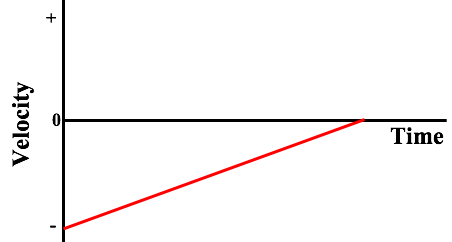A. Is this object speeding up, slowing down, or moving at a constant speed?
B. Is this object experiencing balanced forces or unbalanced forces?

34.  The motion of an object is represented by a velocity-time graph.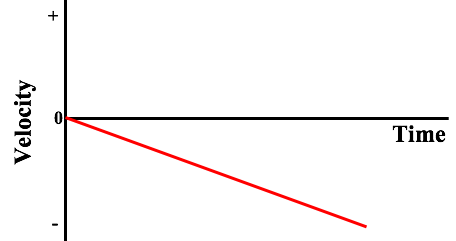A. Is this object speeding up, slowing down, or moving at a constant speed?
B. Is this object experiencing balanced forces or unbalanced forces?

Group #11
35.  The motion of an object is represented by position-time data.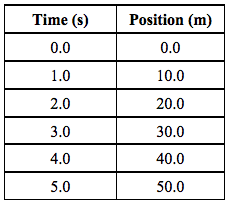A. Is this object speeding up, slowing down, or moving at a constant speed?
B. Is this object experiencing balanced forces or unbalanced forces?

36.  The motion of an object is represented by position-time data.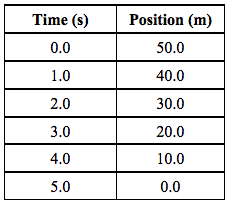A. Is this object speeding up, slowing down, or moving at a constant speed?
B. Is this object experiencing balanced forces or unbalanced forces?

37.  The motion of an object is represented by position-time data.A. Is this object speeding up, slowing down, or moving at a constant speed?
B. Is this object experiencing balanced forces or unbalanced forces?

38.  The motion of an object is represented by position-time data.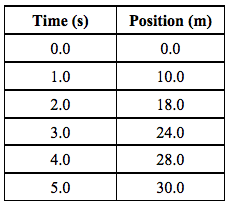A. Is this object speeding up, slowing down, or moving at a constant speed?
B. Is this object experiencing balanced forces or unbalanced forces?

39.  The motion of an object is represented by position-time data.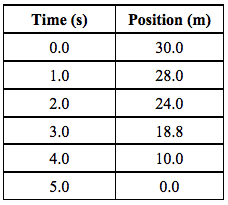A. Is this object speeding up, slowing down, or moving at a constant speed?
B. Is this object experiencing balanced forces or unbalanced forces?

40.  The motion of an object is represented by position-time data.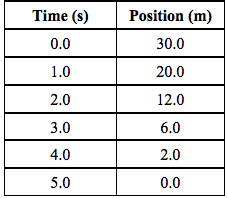A. Is this object speeding up, slowing down, or moving at a constant speed?
B. Is this object experiencing balanced forces or unbalanced forces?

Group #12
41.  The motion of an object is represented by velocity-time data.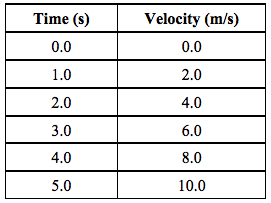A. Is this object speeding up, slowing down, or moving at a constant speed?
B. Is this object experiencing balanced forces or unbalanced forces?

42.  The motion of an object is represented by velocity-time data.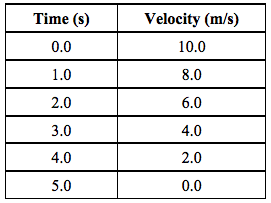A. Is this object speeding up, slowing down, or moving at a constant speed?
B. Is this object experiencing balanced forces or unbalanced forces?

43.  The motion of an object is represented by velocity-time data.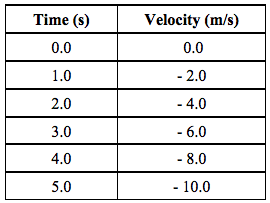A. Is this object speeding up, slowing down, or moving at a constant speed?
B. Is this object experiencing balanced forces or unbalanced forces?

44.  The motion of an object is represented by velocity-time data.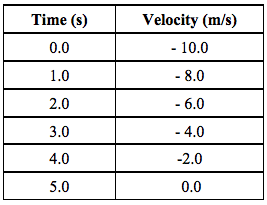A. Is this object speeding up, slowing down, or moving at a constant speed?
B. Is this object experiencing balanced forces or unbalanced forces?